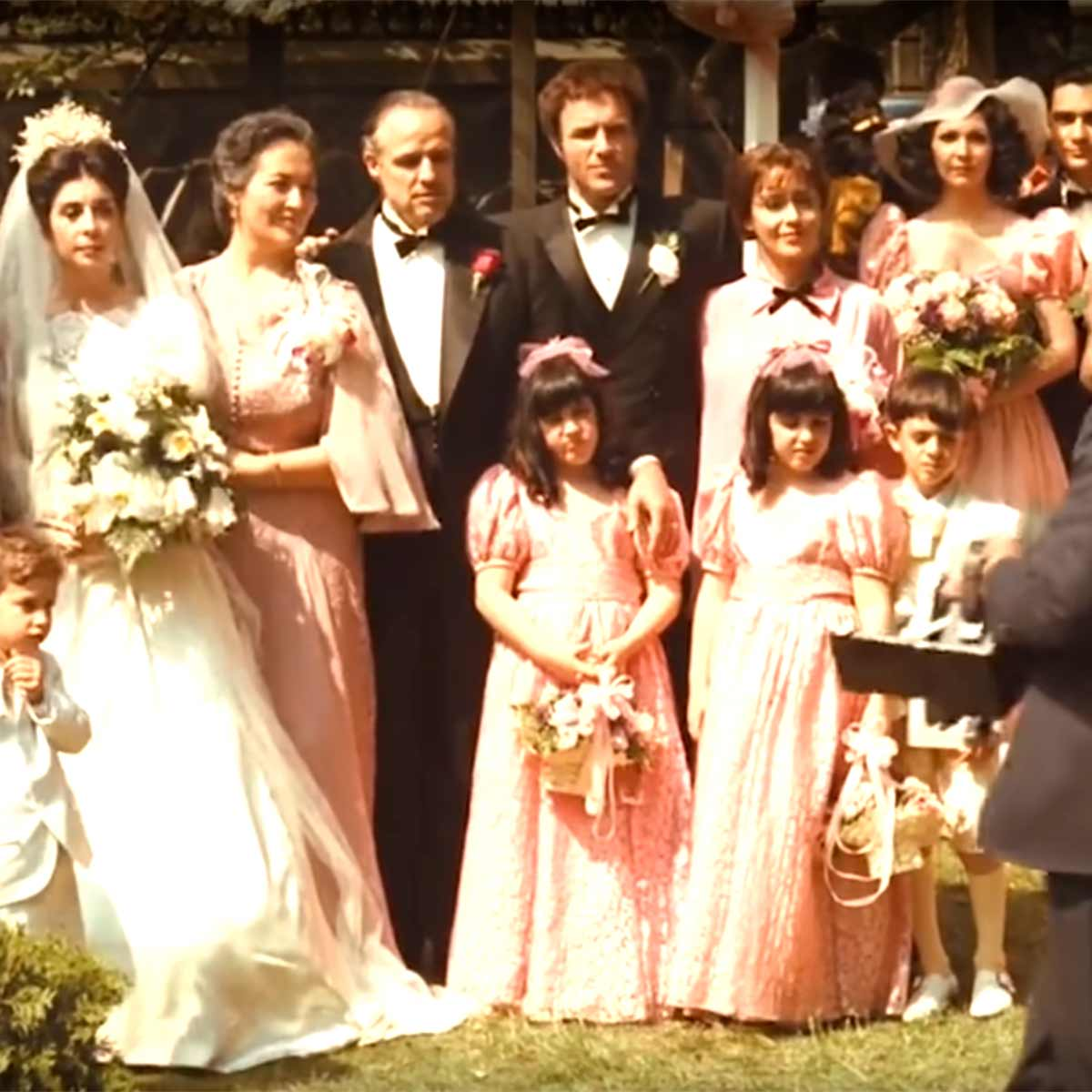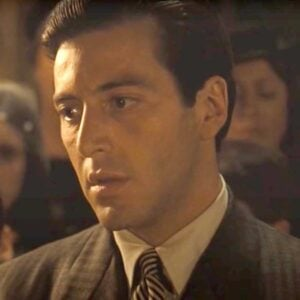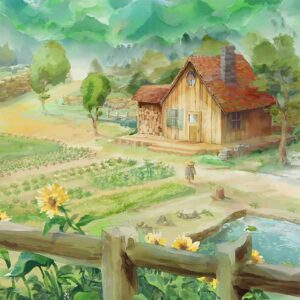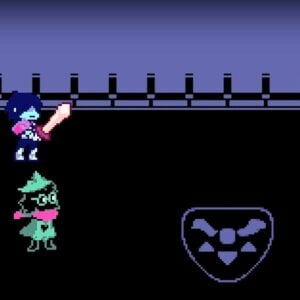# The Godfather Theme (Alternative)

7 July 2021
5
• ##### TARGET LENGTH
00:28
0
(0)

u p s [tea] p s p [yea] p i o [ute]| |
u p s [tea] p s p [ea8] p u Y [yq9]| |
y i O [qa9]| |
y i O [p80]| |
e t o [i0] u o i [i93] u u W [e86]

Rate This Music Sheet:

Average rating 0 / 5. Vote count: 0

No votes so far! Be the first to rate this music sheet.

Thank you for rating this song!

If you have any specific feedback about how to improve this music sheet, please submit this in the box below.

The Godfather Theme (Alternative) is a song by Nino Rota. Use your computer keyboard to play The Godfather Theme (Alternative) music sheet on Virtual Piano. This is an Intermediate song and requires a lot of practice to play well. The recommended time to play this music sheet is 00:28, as verified by Virtual Piano legend, Nova Nine. The song The Godfather Theme (Alternative) is classified in the genre of Songs From Movies on Virtual Piano. You can also find other similar songs using Theme Song, Italy.

• time
user
Rating
ac.
rm.
score
• 2 yWayloft
1066
100
100
7.75

## Other Songs By Nino Rota

•f j l [k6] j [uspl] j [k9] j [ypig] h [f6]| [tsp]||
f j l [k6] j [uspl] j [k8] j [uspf] D [d9]| [ypi]||
d g H [k9]| [ypi]||
d g H [j6]| [usp]|
e p [usp] h [g8] f [usoh] g [g0] f [ufaO] O [pe6]| [usp]||
j j H [h5]| [ywr] h [k5]| [ywrj] g [f8]| [uto] f h f [d9]|
[ypi] d [g9] d [ufaO] [H z x V z x b m]
f j l [k6] j [uspl] j [k9] j [ypig] h [f6]| [tsp]||
f j l [k6] j [uspl] j [k8] j [uspf] D [d9]| [ypi]||
d g H [k9]| [ypi]||
d g H [j6]| [usp]| e p [usp] h [g8] f
[usoh] g [g0] f [ufaO] O [pe6] sfjl[xusp]bmxbmxb

Level: 6
Length: 01:55
Intermediate

#### Nino Rota

•u p s [tp] p s p [ya] p i o [ue] |
u p s [tp] p s p [ew] p u Y [yw] |
y i O [rw] |
y i O [pw] |
e t o [iw] u o i [iw] u u W [ew]

Level: 4
Length: 00:30
Easy

#### Nino Rota

•1 [t8] [ow] [t8] [iy] | 1 [t8] [ow] [t8] W o s D [d1] [s8] D [s8] d s O P [o1] 8 w 8 W o s D [d1] [s8] D [s8] d s o I [i4] 8 W 8 q i O a [d4] 8 q W t | i O a [s1] 5 8 ( w t Y P O [o9] w P O O o o r [t1] 5 8 ( w s s a P ^ 9 q E | d 9 q s O o ( w E o P o [i4] * q W % | [i9] [OW] I [o5] 9 w 9 5 o s D [do1] [s8] D [s8] [di] s O P [o1] 8 w 8 W o s D [do1] [s8] D [s8] [di] s o I [i4] 8 W 8 q i O a [d4] 8 q W t | i O a [s1] 5 8 ( w t Y P O [o9] P O O o o a [s1] 5 8 ( w [ho] [ls] [ZD] [zd] [ls] [ZD] [ls] [zd] [ls] [HO] [JP] [h1] [otw] [tq] [ho] [ls] [ZD] [zd] [ls] [ZD] [ls] [zd] [ls] 8 [ho] [GI] [g4] [i8] [tq] [gi] [HO] [ka] [z4] [d8] [tq] [gi] [HO] [ka] [l1] [sw8] t [st] [DY] [JP] [HO] [ho] [w9] [JP] [HO] [HO] [ho] [ho] [ar] [s1] [tw8] t s s a P ^ 9 q E y i | d 9 q s y O o ( w E o P o i % * q W T % | i [OW] I [o5] 9 w 9 5 o s D [do1] s [D(] s [di] [st] O P [o1] [tw] [tq] o [s8] D [do1] s D s [di] s 8 o I [i4] W 4 i O a [d4] W 4 | i O a [s1] [w8] 1 t [Y(] P O o P O O o [orq] P [s8]
Level: 6
Intermediate

#### Nino Rota

•a f h [3G] f [ruoh] f [6G] f [etus] d [3a] | [wuo] || a f h [3G] f [ruoh] f [5G] f [ruoa] P [6p] | [etu] || p s D [6G] | [etu] || p s D [3f] | [ruo] 0 u [ruo] d [5s] a [ryod] s [7s] a [YIra] Y [30u] [ruo] | f f D [2d] [Q9e] d [2G] [Q9ef] s [5a] [wry] a d a [6p] [etu] p [6s] p [YIra] [D j k Z b n] a f h [3G] f [ruoh] f [6G] f [etus] d [3a] | [wuo] || a f h [3G] f [ruoh] f [5G] f [ruoa] P [6p] | [etu] || p s D [6G] | [etu] | p s D [3f] | [ruo] 0 u [ruo] d [5s] a [ryod] s [7s] a [YIra] Y [30u] oafh[ruok] [x v n x v n]
Level: 6
Length: 02:15
Intermediate

## Related

•7 ( Q Qr r7 0 W Wr r7 ( Q Qr r[YD7]| |7 ( Q Qr r7 0 W Wr r7 ( Q Qr r7| |[D7] ( Q Q[rG]| [f7] 0 [WD] W[rf]| [G7] ( Q Q[rD]| [a7] ( Q Qr| [D7] ( Q Q[rG]| [f7] 0 [WH] W[rG]| [S\$] ^ * *Q| \$ ^ * Q E Q [D7] ( Q Q[rG]| [f7] 0 [WD] W[rf]| [G7] ( Q Q[rD]| [a7] ( Q Qr S [D7] ( [SQ] Q[ra]| [O7] 0 W W[rI]| [a7] ( Q Qr| 7 ( Q r Y I [I7]aD( Q Q[rG]| [f7]Gf0 [WD] W[rf]| [G7] ( Q Q[rD]| [a7] ( Q Qr| [I7]aD( Q Q[rG]| [f7] 0 [WH] W[rG]| [I\$]PS^ * *Q| \$ ^ * Q E Q [I7]aD( Q Q[rG]| [f7]Gf0 [WD] W[rf]| [G7] ( Q Q[ra]| [H7] 0 [WG] W[rf]| [D7] ( [SQ] Q[ra]| [O7] 0 W W[rI]| [a7] ( Q ( r Q Y r I Y a P [rk] Y I a I [YJ] [rH] u O a O [uf] [rG] Y I a I [YD] [SQ] E T I [aT] [SE] [rD] Y I a [OI] Y [rf] u [OD] a [fO] u [rG] Y I a IG[YJ]k[QC] E T I T E [rk] Y I a I [YJ] [rH] u O a [fO] u [rG] Y I a I [YH] [QJ] E T I T [GE] [k(] W r Y r [WL] [J(] Q E T [GE] Q [rk] Y I a I Y r Y I a I Y [rG7]DSaGDSaGDSa[D7]SaIDSaIDSaI[aW%]POYaPOYaPOY[O30]IurOIurOIur[rQ7]rY

Level: 5
Length: 01:54
Intermediate

#### Eric Barone

•e tee|e te0 w0 e tee|e te0 w0 e tee|e te0 w0 e|0 w0e|0 w0 [ute]|[yre]|[ute]| [te] [yre]|[re]|[ute]|0 w0 [ute]|[yre]|[ute]| [te] [yre]|[re]|[ute]|| [e0]|t|0| e [y0]|u|0|w| [e0]| yt[r0]|[tq]| [eQ]|9|e te[e9]| [e0]|t|[e0]| u [eI9]|y|[e9]|| [uqp]||e o t i [yw]||q|| [tie0]||u 0 y [re]||w|| [wt]||q r 8 r [qe]||w|| [ute]|[yre]|[ute]| [te] [yre]|[re]|[ute]|0 w0 [ute]|[yre]|[ute]| [te] [yre]|[re]|[ute]
Level: 5
Length: 00:52
Intermediate

#### John Williams

•[sf]| [sg]| [qp] s h [th]|[ih]|[oh]|h| s|f|[o0] s d [td]|[yd]|d|d| f|d|[sq]|[ts]|[si]|[so]|s| k|l|[spkY]| j k j| f| [sf]|[sg]|[p9] s h [he]|[th]|[yh]|h| s|f|[o0] s d [ed]|[td]|[yd]|[od]| f|d [sq] e y t|i|o|[wrf9]|g|[rdW9]|s|[te0]|s||d H l z||| f|d|[s9] q e t| o|[wf]|g|[qd]|s|t| s||| [e4] t y o||| [w3] t y||| [t4]|8|q|w| | [tea(]||| [te(]||| [p9] s h|e|t|y| t|9| [o0] s d e|t|y|o| | [sq] e y t|i| [wrf9]| [rdW9]| [te0]| |[ytW9]||| y i p s||5| %| 6|0|r|t|o|a|s|h|k|l|v
Level: 6
Length: 01:21
Intermediate